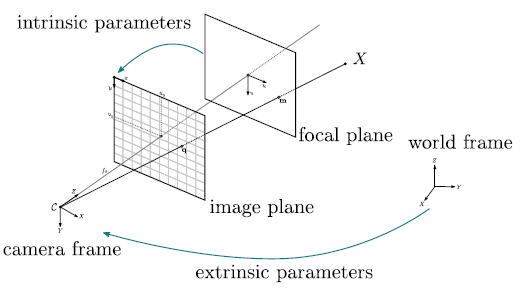# 清晰說明針孔相機的內部參數與外部參數矩陣

## 成像的原理$$\begin{bmatrix} u \\ v # \end{bmatrix}\ \begin{bmatrix} m_uf & 0 & m_ut_u \\ 0 & m_vf & m_vt_v \\ 0 & 0 & 1 \end{bmatrix}\ \begin{bmatrix} X \\ Y \\ Z \end{bmatrix}\$$$$\begin{bmatrix} u \\ v # \end{bmatrix}\ \begin{bmatrix} m_uf & s & m_ut_u \\ 0 & m_vf & m_vt_v \\ 0 & 0 & 1 \end{bmatrix}\ \begin{bmatrix} X \\ Y \\ Z \end{bmatrix}\$$

## 外部參數矩陣## 總結

• 內部參數矩陣：處理相機座標系到影像座標系的投影
• 外部參數矩陣：處理世界座標系到相機座標系的座標轉換